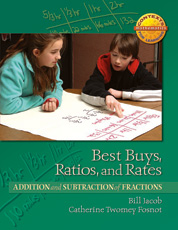# Best Buys, Ratios, and Rates

## Addition and Subtraction of Fractions

By Catherine Twomey Fosnot, William Jacob

Best Buys, Ratios, and Rates: Addition and Subtraction of Fractions is one of five units in the Contexts for Learning Mathematics’ Investigating Fractions, Decimals, and Percents (4–6)

The focus of this unit is the development of equivalence of fractions, proportional reasoning, and rates. It begins with a comparison of the cost of cat food at two stores: Bob’s Best Buys where it is on sale, \$15 for 12 cans, and Maria’s Pet Emporium where it is on sale, \$23 for 20 cans. Several important ideas and representations develop as students explore this problem,
...

### Paperback

In Stock

List Price: \$31.25

Web/School Price: \$25.00

##### PART OF THE SERIES

The Context for Learning Mathematics Series

#### Full Description

Best Buys, Ratios, and Rates: Addition and Subtraction of Fractions is one of five units in the Contexts for Learning Mathematics’ Investigating Fractions, Decimals, and Percents (4–6)

The focus of this unit is the development of equivalence of fractions, proportional reasoning, and rates. It begins with a comparison of the cost of cat food at two stores: Bob’s Best Buys where it is on sale, \$15 for 12 cans, and Maria’s Pet Emporium where it is on sale, \$23 for 20 cans. Several important ideas and representations develop as students explore this problem, among them finding ways to determine the cost of a common numbers of cans for comparison and the use of the ratio table to represent their proportional reasoning about the context. The development of the ratio table is further supported in the next investigation as students work to determine the cost of several different amounts of bird seed sold by weight. As the unit progresses, proportional reasoning is once again the focus as students develop recipes for a variety of containers, using the recipe of Maria’s gourmet puppy snack mix.

In the second week the double number is introduced for computation as students investigate the readings on a farm truck’s gas tank over the course of trips to several neighboring farms to pick up produce. A trip across the Pennsylvania Turnpike is also explored.

This unit also includes several minilessons for addition and subtraction of fractions. Strings of related problems are used initially using money and clock models. Double number lines are introduced later in the unit to enable students to develop generalizable, strategies for addition and subtraction. This model supports students to choose a common multiple (or factor) to work with as well as further opportunities to explore equivalent fractions.

Note: The context for this unit assumes that your students have had prior experience with fractions and their relationship to division with whole numbers. If this is not the case, you might find it helpful to first use the units Field Trips and Fund-Raisers.